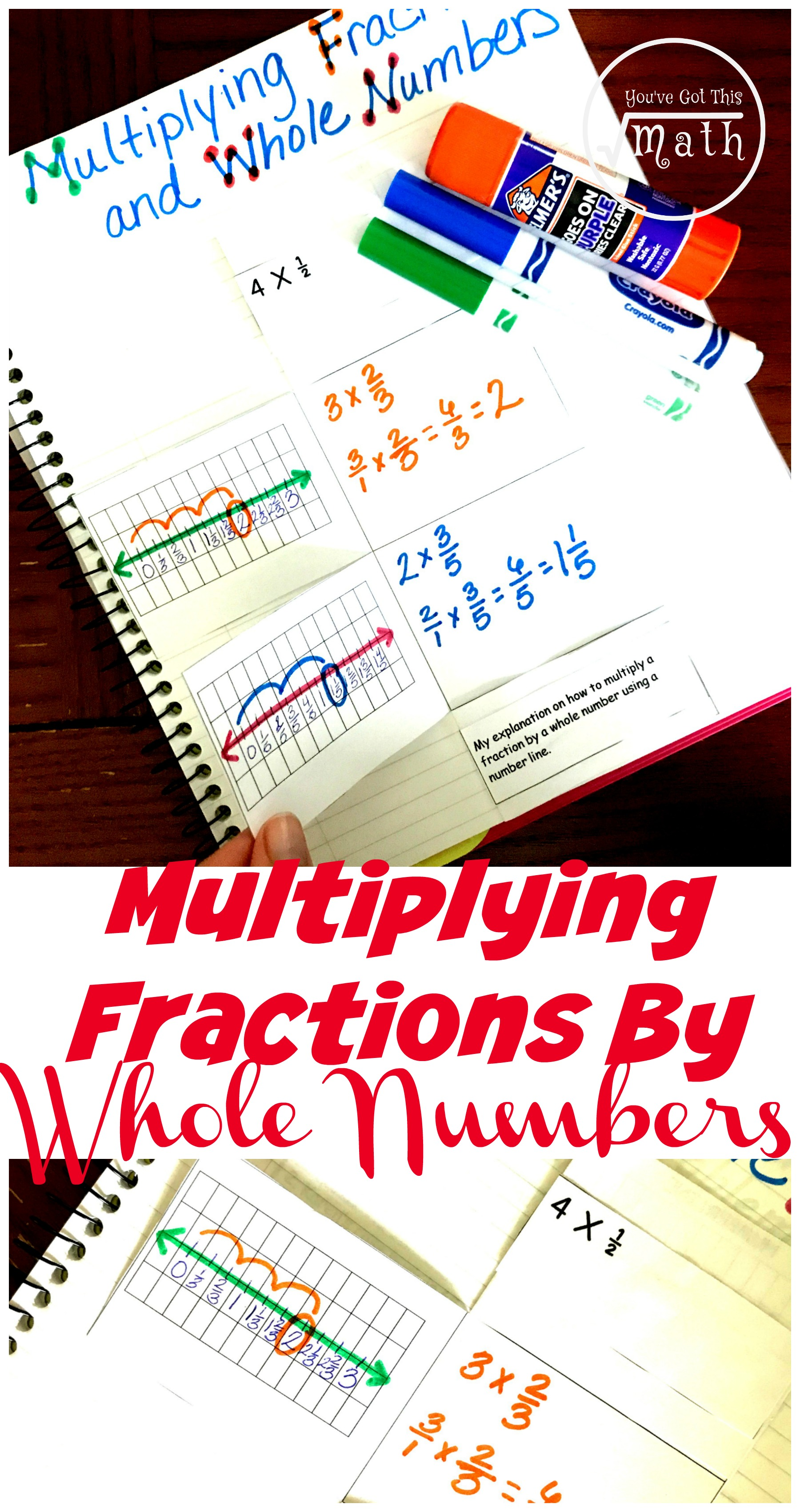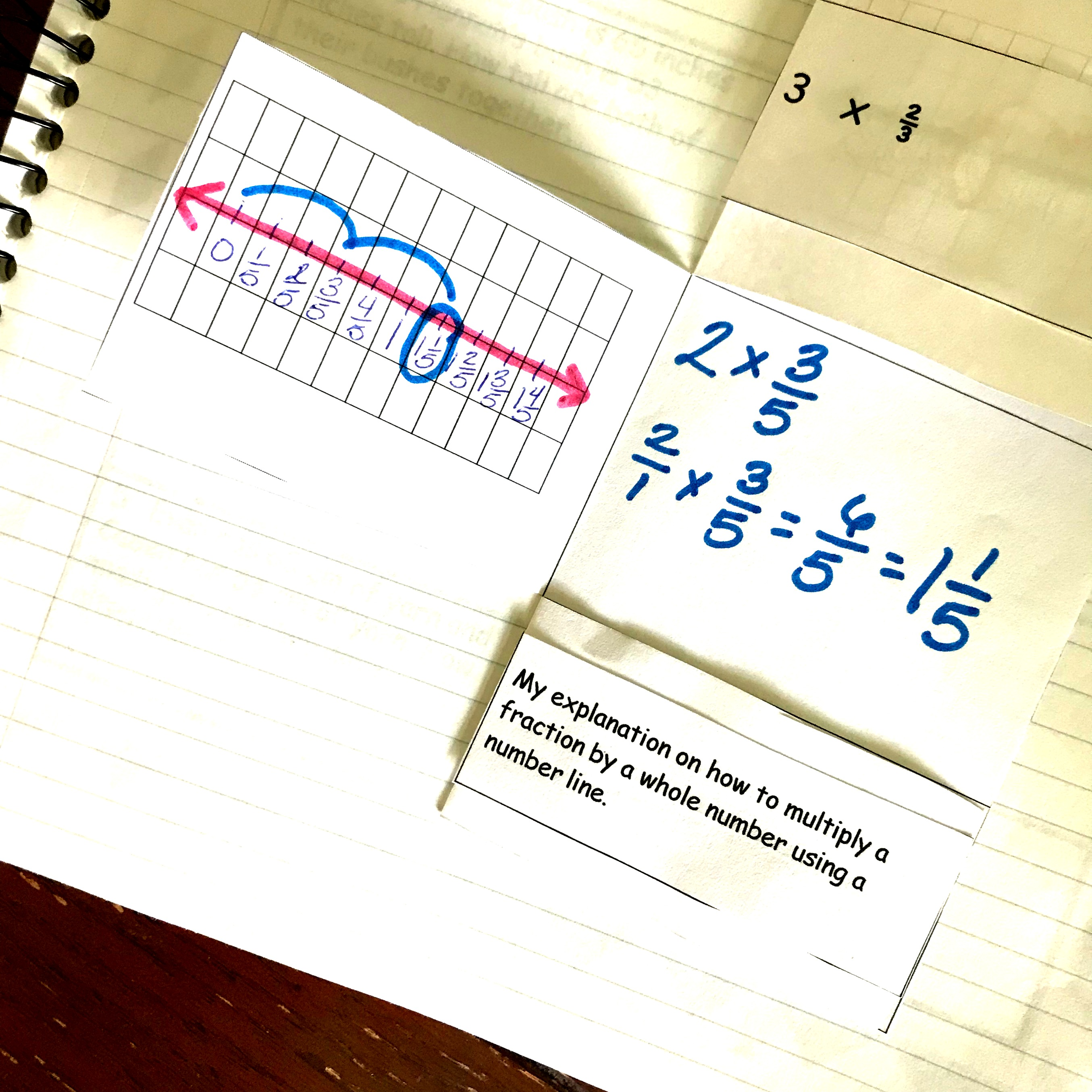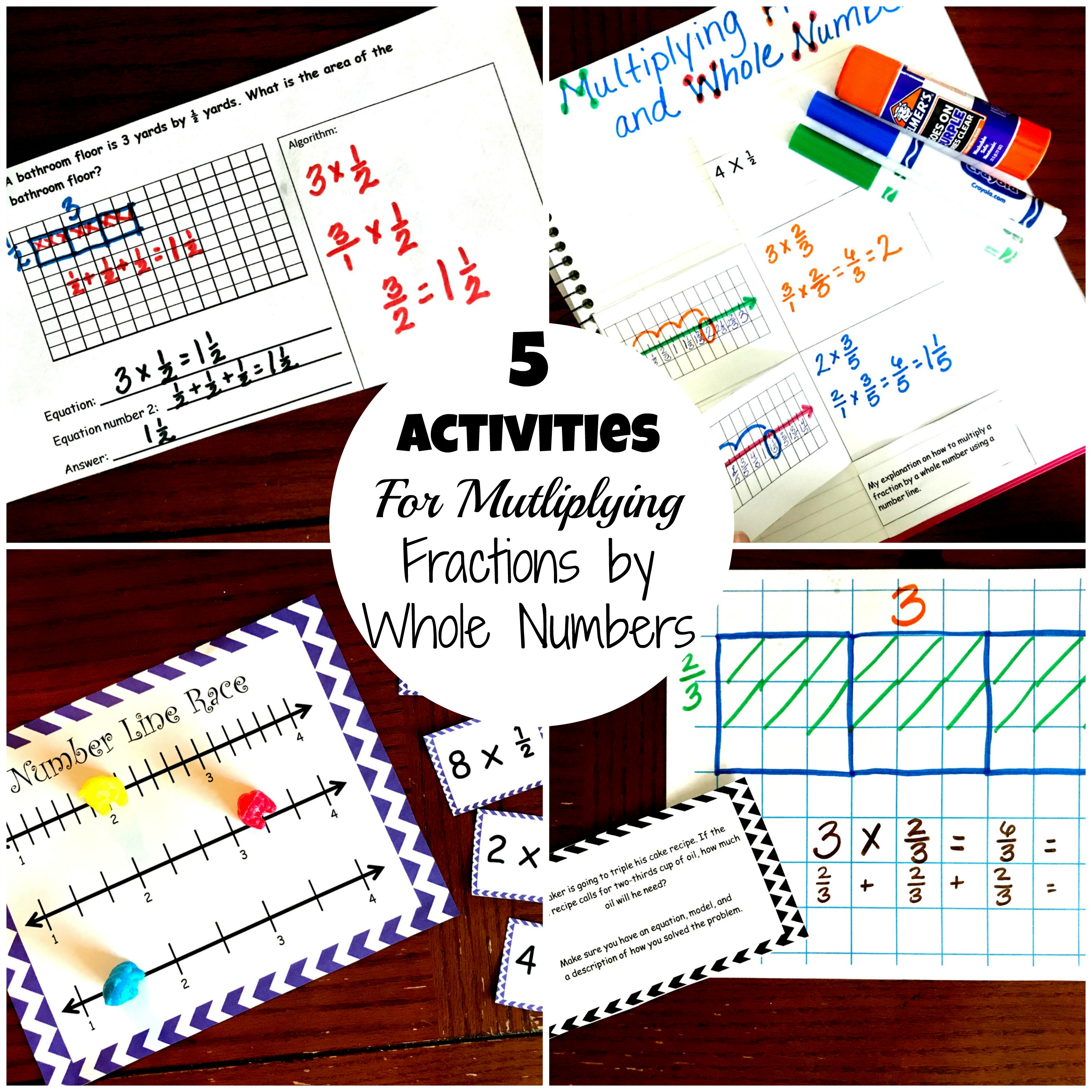Home » Math » FREE Multiplying Fraction Activity To Teach Whole Numbers by Fractions

FREE Multiplying Fraction Activity To Teach Whole Numbers by Fractions

This multiplying fraction activity will help students understand what how to multiply fractions by whole numbers using the number line and an algorithm.You can get this FREE printable by clicking on the blue button at the VERY end of the post!

One of my favorite subjects to teach as a fifth-grade teacher was fractions. I love teaching fractions, and even crazier, I love teaching them on a number line. It is so neat and organized and you can visually see what you are doing. It makes me happy to see all those little factions lined up!!

Teaching fractions on a number line accomplish something I’m a firm believer in. I believe that students should understand why they are doing something and not just know how to do an algorithm.

I was never good at math while I was in school, but the process of teaching with manipulatives, decomposing numbers, and drawing models has brought an understanding of math I never would have thought possible. I actually enjoy it now.

So to help you are your students understand fraction multiplication, this post contains a FREE multiplying fractions activity for you use to review or begin teaching multiplying fractions by a whole number using a number line.

Prep-Work

1. Print off the interactive notebook.
2. Next, have your children glue the two middle rows into their math journals.
3. Now, have them cut on the dotted lines.
4. Finally, gather up some pencils and markers so they can complete this interactive notebook with you.

Multiplying Fraction Activity

In upper grades, students are very familiar with multiplying. Hopefully, at this point, your students understand that multiplication is a quick way of figuring out how many of a certain item you have when that item has been separated into equal groups. They should recognize that they could solve the multiplication problem by skip counting or repeated addition, but it is not time efficient.

If they understand this, then multiplying fraction on a number line will not be hard for them.

Let’s say the problem in 2 x 3/5.The students begin by creating a number line at 0 and then adding fifths to each increment.

Next, they start “hopping” by three fifths…..and they do this two times. It is that simple.

Example 2:In this problem, we are multiplying 2/3 by 3. So we will once again use our denominator, 3, and create fractions on the line in increments of 1/3. Once our number line is filled out, we will “hop” two-thirds for three times. We will end with the number two……the answer.

So if you have a student struggling with fractions, try getting away from the algorithm and finding manipulatives, a number line, or models and let them explore. You may be amazed at the understanding that begins to develop!!

Want more multiplication practice?